﻿ PipeLay > Technical Notes > Technical Note 4 - Axis Systems & Conventions > Global Axis System

# Global Axis System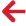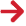PipeLay uses a number of axis systems. Of these, the most important are the global or XYZ axes. These are the axes in which many (though by no means all) coordinates  and loads are input, they are the axes in which the total system equations of motion are assembled and solved internally by PipeLay, and they are finally the axes in which pipeline motions and reactions at restrained locations are output. In this system, the global X axis is vertical, with the global Y and Z axes lying in a horizontal plane such that the system is right-handed. Zero datum is located at X=0, so for example, if you are using the default flat seabed profile, then the global Y and Z-axes both lie on the seabed. See the figure below.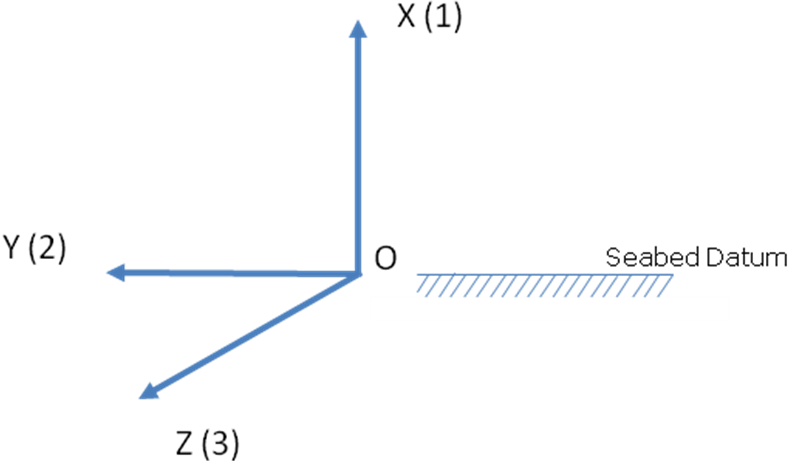PipeLay Global Axes

This convention is consistent with all of the other Wood Group software suites, for example, Flexcom. This convention may contrast with the conventions used in other finite element or draughting packages, so you should take care when creating PipeLay models to ensure you are following the correct convention.

Global Degrees of Freedom

The independent components required to define the movement or displacement of a point on a structure are known as its degrees of freedom, or DOFs for short. A point on a pipeline requires 6 DOFs to define its movement – three translations (one vertical, two horizontal) and three rotations. In the PipeLay convention, a movement or displacement in the global X direction is denoted a DOF 1 translation, a displacement in global Y is a DOF 2 translation, while DOF 3 is used for a translation in the global Z direction.

The rotational displacements are denoted DOFs 4-6 in PipeLay. Explaining what these represent physically is a little more complicated than the translations case. Taken together, they represent the rotation in 3D space of a section of pipeline from its initial orientation. You will no doubt be aware that finite (non-infinitesimal) 3D rotations cannot be represented as vectors, since the addition of such vectors is non-commutative, that is, the order in which the rotations are taken affects the result. In PipeLay this problem is handled by means of a consistent 3D kinematics formulation based on the correspondence between a specially defined rotation vector and a transformation matrix. Manipulation of the rotation is undertaken by means of operations on the transformation matrices. (You will note that as required matrix multiplication is non-commutative.)

The cornerstone of this formulation is the definition of the rotation vector referred to above. The full details are omitted here, but, in summary, a rotation is represented by a vector such that i) the magnitude of the vector represents the magnitude of the rotation, and ii) the direction of the vector represents the axis of rotation. DOFs 4-6 in PipeLay are the components of this rotation vector. This means that in general they do not represent individual rotations about the global X, Y and Z axes.

While this is true in general, in many cases the situation is somewhat simplified. Firstly, in an analysis where the structure and environment are in either the XY or XZ plane, then in this 2D case there is obviously only one rotation component active (for example, DOF 6 if structure and environment are in the vertical XY plane, as illustrated in the figure below).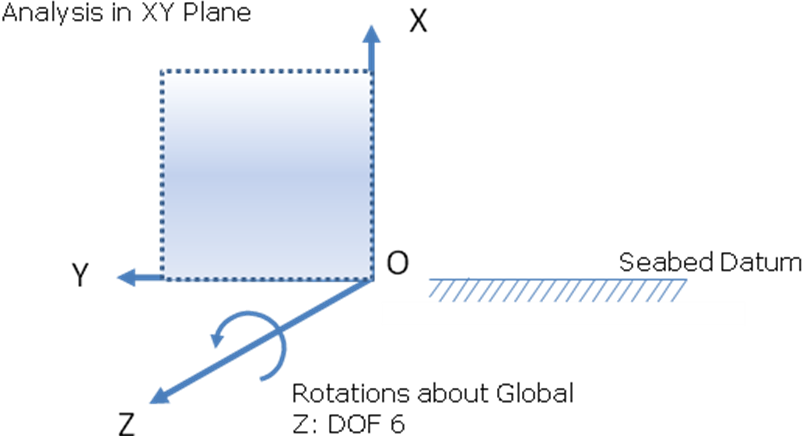2D Analysis in XY Plane

Secondly, in a 3D analysis, the individual components will represent individual rotations about the global axes if the overall rotation is 'small', say less than 10°. See the figure below, which shows incidentally the positive sense of these rotations.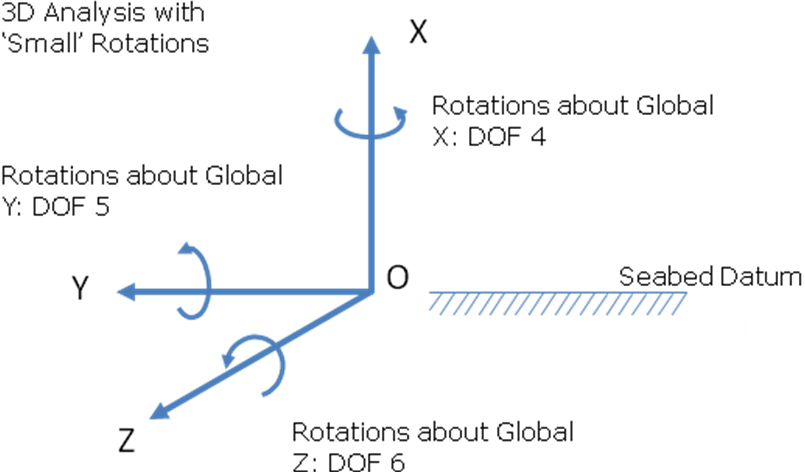3D Analysis with Small Rotations

The only time the PipeLay user really needs to be aware of these rotation definitions and conventions is if you are applying non-zero rotational restraints or boundary conditions in the Model component. (The Model component is the subject of another article.) It is important if specifying displacements in DOFs 4-6 to understand the significance of these degrees of freedom, as described above, and to be clear that they do not in the most general case represent individual rotations about the global axes. In particular, this means you should be wary of specifying only 1 or 2 rotation components (that is, 1 or 2 only of DOFs 4-6) where large rotations are involved, and you should only do so if you understand exactly the effect of what you are doing. Of course, if you are not specifying non-zero rotational boundary conditions, which will be the case in the overwhelming majority of analyses, then this is not an issue.

## User-Defined Axes

In addition to the global and other pre-defined axes in PipeLay, the program does provide the option for you to define an arbitrary or ‘local’ system of axes. There is an component you use for this purpose. The local axes are defined by inputting, as components in the global axes, two of the three vectors defining the axes, namely the local x-axis and y-axis. These must be orthogonal, and provided they are, PipeLay works out the component of the local z-axis using the right-hand rule. For example, in the simplified specification below where the local axes simply represent a rotation of the global axes about global X, appropriate inputs for the local x-axis would be (1, 0, 0) while the local y-axis could be defined as (0, cos θ, sin θ).User-defined or Local Axes

One of the places you can employ a user-defined axis system is in defining boundary conditions in the component, and again you have the option (in certain circumstances) of defining rotational boundary conditions in this way. The same comments made previously about understanding the significance of displacements in DOFs 4-6 apply with equal validity to local axes. Defining rotational constraints in local axes would in general represent an uncommon or unusual specification.